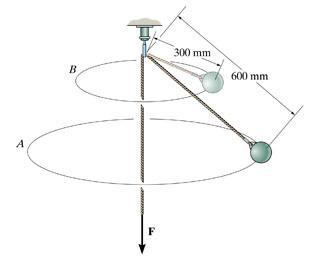# Angular Momentum of a rotating mass

• grassstrip1
In summary, the conversation discusses a problem involving a rotating bob on a horizontal circular path. The initial and final angular momentums and velocities are calculated using the equations L = Iω and v = rω, but the resulting answer does not match the expected one due to the changing angle theta. It is suggested to use Newton's laws to find theta and then apply it to the conservation of angular momentum equation. Additionally, it is noted that gravity plays a role in the motion of the rotating bob.

#### grassstrip11. Homework Statement

When the 3.2-kg bob is given a horizontal speed of 1.5 m/s, it begins to rotate around the horizontal circular path A. The force F on the cord is increased, the bob rises and then rotates around the horizontal circular path B. (picture included)

L = I ω v = rω

## The Attempt at a Solution

The solution from the book goes into a long procedure with summation of forces to find the angle etc. I tried this

Since there is no moment about the z axis angular momentum is conserved about the z axis. I1ω1 = I2ω2
For a particle I= mr2 so initially, I = (3.2)(0.6sinθ)2 and after I = (3.2)(0.3sinθ)2. For ω, initially it is (0.6)(sinθ)(1.5m/s) at the end is is (0.3sinθ)(v2)

Setting these equal to each other and solving gives v=12m/s But the answer is 2m/s Not sure where I am going wrong. Thank you!

#### Attachments

You don't seem to mark theta on your diagram. I assume it is the angle the chord makes with the z axis. If so, it will not be the same in both positions.
As the tension increases, the "orbit" becomes more horizontal and theta changes.

Ah that would explain it. I think i need to find theta using Newton's laws. Then I can apply it to the conservation of angular momentum.

Thank You.

Remember that the reason the ball is not just spinning with the chord horizontal, is gravity. The tension in the chord is providing the opposition to gravity as well as centripetal force.